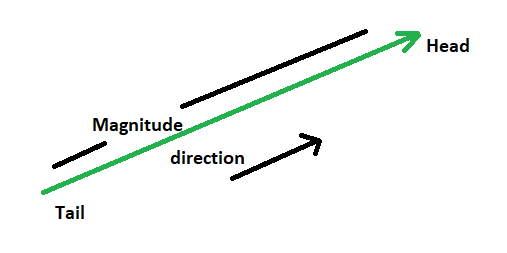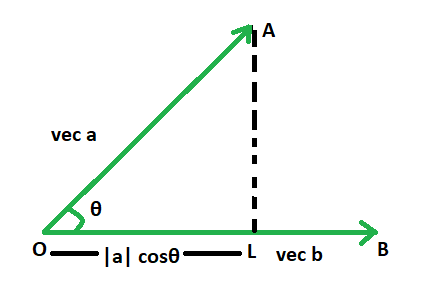Open in App
Not now

# Vector Projection Formula

• Last Updated : 29 Dec, 2022

A vector is a two-dimensional object that has magnitude as well as direction. A vector can be seen geometrically as a directed line segment with an arrow indicating the vector’s direction and a length equal to the vector’s magnitude. From the tail to the head is the direction of the vector. Two vectors are the same if they have the same magnitude and direction. This means that if we translate a vector to a new point (without rotating it), the vector we derive is the same as the one that we started with.## Projection Vector

The length of a given vector’s shadow cast over another vector is the vector projection of one vector over another vector. It’s calculated by multiplying the magnitude of the two vectors by the cosecant of the angle between them. A scalar value is the outcome of a vector projection formula.

### Formula

The dot product of vector a and vector b, divided by the magnitude of vector b, is the projection vector formula in vector algebra for the projection of vector an on vector b. The dot product’s result is a scalar value, and the vector b’s magnitude is also a scalar value. As a result, the projection vector answer’s magnitude and argument are both scalar values in the direction of vector b.

Projection of Vector a on Vector b =### Derivation of Vector ProjectionFrom the right triangle OAL , cos θ = OL/OA

OL = OA cos θ

⇒ OL =cos θ

OL is the projection vector of vector a on vector b.

We know,OL =Hence proved.

## Sample Problems

Question 1. Find the projection of the vectorand.

Solution:

Here,.

We know, projection of Vector a on Vector b =Question 2. Find the projection of the vectorand.

Solution:

Here,We know, projection of Vector a on Vector b =Question 3. Find the projection of the vectorand.

Solution:

Here,We know, projection of Vector a on Vector b =Question 4. Find the projection of the vector 2\hat i – 6\hat j + \hat k and 8\hat i – 2\hat j + 4\hat k .

Solution:

Here,We know, projection of Vector a on Vector b =Question 5. Find the projection of the vector 2\hat i – \hat j + 5\hat k and 4\hat i – \hat j + \hat k.

Solution:

Here,We know, projection of Vector a on Vector b =My Personal Notes arrow_drop_up